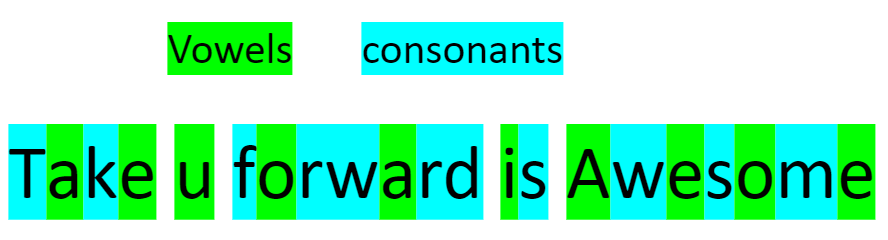# Count number of vowels, consonants, spaces in String

Problem Statement: Given a string, write a program to count the number of vowels, consonants, and spaces in that string.

Examples:

```Example 1:
Input: string str=”Take u forward is Awesome”
Output:
Vowels: 10
Consonants: 11
White spaces: 4
Explanation:Example 2:
Input: string str=”India won the cricket match”
Output:
Vowels: 8
Consonants: 15
White spaces: 4
```

### Solution

Disclaimer: Don’t jump directly to the solution, try it out yourself first.

Approach:

• Convert the given string into lowercase for uniformity while counting vowels,consonants,whitespaces.
• This can be done by using towlower() and .toLowerCase() in C++ and Java respectively.
• Traverse the entire modified string by applying the following conditions:

If any vowel then increment the vowel counter

Else if any consonant then increment the consonant counter else if any whitespace then increment the whitespace counter

Code:

## C++ Code

``````#include<bits/stdc++.h>

using namespace std;
int solve(string str, int length) {
int vowels = 0, consonants = 0, whitespaces = 0;
for (int i = 0; i < length; i++) // converting given string to lowercase
{
str[i] = towlower(str[i]);
}
for (int i = 0; i < length; i++) {
if (str[i] == 'a' || str[i] == 'e' || str[i] == 'i' || str[i] == 'o' || str[i] == 'u')
vowels++;
else if (str[i] >= 'a' && str[i] <= 'z')
consonants++;
else if (str[i] == ' ')
whitespaces++;
}

cout << "Vowels: " << vowels << "\n";
cout << "Consonants: " << consonants << "\n";
cout << "White Spaces: " << whitespaces << "\n";

}
int main() {
string str = "Take u forward is Awesome";
int length = str.length();
solve(str, length);
return 0;
}
``````

Output:

Vowels: 10
Consonants: 11
White Spaces: 4

Time Complexity: O(n), n is the length of string

Space Complexity: O(1)

## Java Code

``````public class tUf {
public static void solve(String str, int length) {
int vowels = 0, consonants = 0, whitespaces = 0;
str = str.toLowerCase(); // converting given string to lowercase
for (int i = 0; i < length; i++) {
char ch = str.charAt(i);
if (ch == 'a' || ch == 'e' || ch == 'i' || ch == 'o' || ch == 'u')
vowels++;
else if (ch >= 'a' && ch <= 'z')
consonants++;
else if (ch == ' ')
whitespaces++;
}

System.out.println("Vowels: " + vowels);
System.out.println("Consonants: " + consonants);
System.out.println("White spaces: " + whitespaces);
}
public static void main(String args[]) {
String str = "Take u forward is Awesome";
int length = str.length();
solve(str, length);
}
}
``````

Output:

Vowels: 10
Consonants: 11
White Spaces: 4

Time Complexity: O(n), n is the length of string

Space Complexity: O(1)